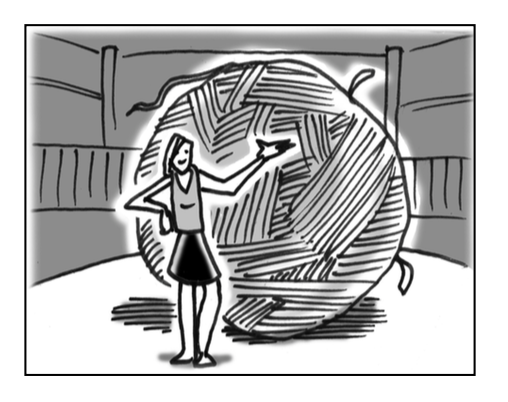### Home > INT2 > Chapter 12 > Lesson 12.1.1 > Problem12-11

12-11.
1. 12-11.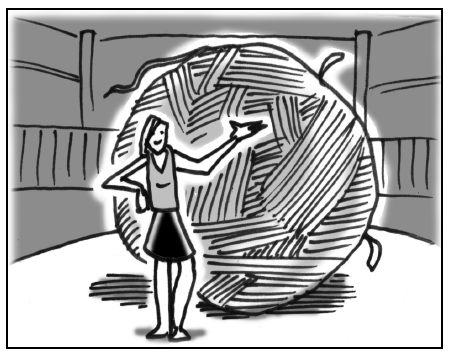Cawker City, Kansas, claims to have the world’s largest ball of twine. Started in 1953 by Frank Stoeber, this ball has been created by wrapping more than 1300 miles of twine. In fact, this giant ball has a circumference of 40 feet. Homework Help ✎

1. Assuming the ball of twine is a sphere, what are the surface area and volume of the ball of twine?

2. The neighboring town wants to create a ball of twine with double the volume of Cawker City’s ball of twine. What will be the circumference of this ball of twine?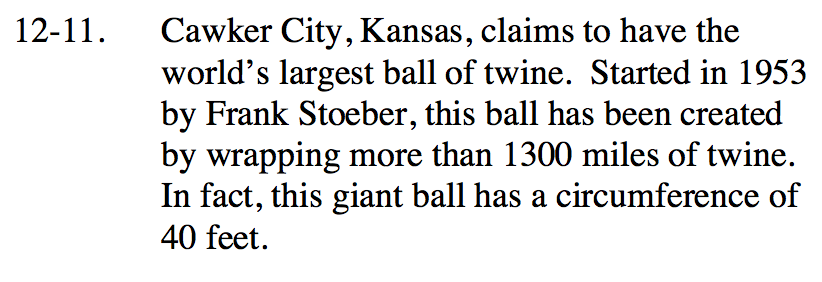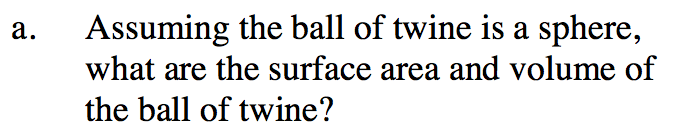The equation for circumference is 2πr.

Use the radius to solve for surface area and volume.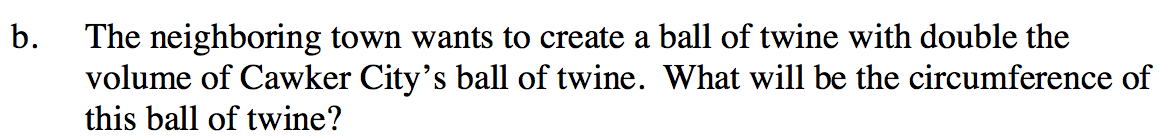If the volume is increased by a factor of 2 times, then circumference will be increased by a factor of 21/3.